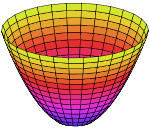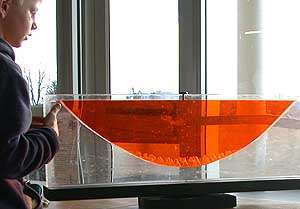# Rotating furnaceAxially symmetrical paraboloid. The inside surface is concave

A rotating furnace is a device for making solid objects which have concave surfaces that are segments of axially symmetrical paraboloids. Usually, the objects are made of glass. The furnace makes use of the fact, which was known already to Newton, that the centrifugal-force-induced shape of the top surface of a spinning liquid is a concave paraboloid, identical to the shape of a reflecting telescope's primary focusing mirror.

Paraboloids can be used in various ways, including (after being silvered) as primary mirrors in reflecting telescopes and solar cookers.

## DesignParabolic shape formed by a liquid surface under rotation. Two liquids of different densities completely fill a narrow space between two sheets of plexiglass. The gap between the sheets is closed at the bottom, sides and top. The whole assembly is rotating around a vertical axis passing through the centre

The furnace includes a mechanism that rotates an open-topped container at constant speed around a vertical axis. A quantity of glass sufficient to make the mirror is placed in the container, heated until it is completely molten, and then allowed to cool while continuing to rotate until it has completely solidified. When the rotation is stopped, the glass is solid, so the paraboloidal shape of its top surface is preserved. This process is called spin casting.

The same process can be used to make a lens with a concave paraboloidal surface. The other surface is shaped by the container that holds the molten glass acting as a mould. Lenses made this way are sometimes used as objectives in refracting telescopes.

The word spin is frequently used in this context without implying that the rotation is rapid. Making a mirror with a focal length of five metres, for example, requires a rotation speed less than ten revolutions per minute. Since the speed is low, accurate dynamic balancing of the rotating components is not needed.

The axis of rotation becomes the axis of the paraboloid. It is not necessary for this axis to be in the centre of the container of glass, or even for it to pass through the container. By placing the container away from the axis, off-axis paraboloidal segments can be cast. This is done in the making of very large telescopes which have mirrors consisting of several segments.

## Mathematical model

### Rotation speed and focal length

The focal length of the paraboloid is related to the angular speed at which the liquid is rotated by the equation:, whereis the focal length,is the rotation speed, andis the acceleration due to gravity. They must be in compatible units so, for example,can be in metres,in radians per second, andin metres per second-squared. The angle unit inmust be radians. 1 radian per second is about 9.55 rotations per minute (RPM). On the Earth's surface,is about 9.81 metres per second-squared. Putting these numbers into the equation produces the approximation:, whereis the focal length in metres, andis the rotation speed in RPM.

## Uses

Generally, a spin-cast paraboloid is not sufficiently accurate to permit its immediate use as a telescope mirror or lens, so it is corrected by computer-controlled grinding machines. The amount of grinding done, and the mass of glass material wasted, are much less than would have been required without spinning.

Spin casting can also be used, often with materials other than glass, to produce prototype paraboloids, such as spotlight reflectors or solar-energy concentrators, which do not need to be as exactly paraboloidal as telescope mirrors. Spin casting every paraboloid that is made would be too slow and costly, so the prototype is simply copied relatively quickly and cheaply and with adequate accuracy.

Liquid mirror telescopes have rotating mirrors that consist of a liquid metal such as mercury or a low-melting alloy of gallium. The mirrors do not solidify, but are used while liquid and rotating. The rotation shapes them into paraboloids that are accurate enough to be used as primary reflectors in telescopes. No correction of the shape is necessary. Spin-cast glass mirrors need correction because of distortions that arise during and after solidification.